PSEB 9th Class Maths MCQ Chapter 15 Probability

Punjab State Board PSEB 9th Class Maths Book Solutions Chapter 15 Probability MCQ Questions with Answers.

PSEB 9th Class Maths Chapter 15 Probability MCQ Questions

Answer each question by selecting the proper alternative from those given below each question to make the statement true:

Question 1.
When a balanced die is thrown, the probability of getting 3 is …………….. .
A. $$\frac{1}{3}$$
B. $$\frac{1}{2}$$
C. $$\frac{1}{4}$$
D. $$\frac{1}{6}$$
D. $$\frac{1}{6}$$Question 2.
A card is drawn at random from a well shuffled pack of cards. The probability of that card being a king is …………………. .
A. $$\frac{1}{52}$$
B. $$\frac{1}{26}$$
C. $$\frac{1}{13}$$
D. 1
C. $$\frac{1}{13}$$

Question 3.
A card is drawn at random from a well shuffled pack of cards. The probability of that card being a card other than picture cards is ……………….. .
A. $$\frac{4}{13}$$
B. $$\frac{10}{13}$$
C. $$\frac{3}{13}$$
D. $$\frac{1}{13}$$
B. $$\frac{10}{13}$$Question 4.
When an unbiased coin is tossed thrice, the probability of receiving three heads is ………………… .
A. $$\frac{1}{8}$$
B. $$\frac{1}{4}$$
C. $$\frac{1}{2}$$
D. $$\frac{3}{8}$$
A. $$\frac{1}{8}$$

Question 5.
When three unbiased coins are tossed simultaneously, the probability of receiving exactly one tail is ………………… .
A. $$\frac{1}{8}$$
B. $$\frac{1}{2}$$
C. $$\frac{1}{4}$$
D. $$\frac{3}{8}$$
D. $$\frac{3}{8}$$Question 6.
When a balanced die is thrown, the probability of receiving an even number is ………………… .
A. $$\frac{1}{6}$$
B. $$\frac{5}{6}$$
C. $$\frac{1}{2}$$
D. $$\frac{1}{4}$$
C. $$\frac{1}{2}$$

Question 7.
When a balanced die is thrown, the probability of receiving a prime number is ……………….. .
A. $$\frac{2}{3}$$
B. $$\frac{3}{4}$$
C. $$\frac{1}{3}$$
D. $$\frac{1}{2}$$
D. $$\frac{1}{2}$$Question 8.
When two balanced dice are thrown simultaneously, the probability of getting the total of numbers on dice as 9 is ………………. .
A. $$\frac{1}{9}$$
B. $$\frac{1}{6}$$
C. $$\frac{1}{3}$$
D. $$\frac{1}{12}$$
A. $$\frac{1}{9}$$

Question 9.
Out of 100 days, the forecast predicted by the wheather department proved to be true on 20 days. Chosen any one day from these 100 days, the probability that the forecast proved to be false is ………………… .
A. $$\frac{1}{3}$$
B. $$\frac{1}{4}$$
C. $$\frac{3}{4}$$
D. $$\frac{4}{5}$$
D. $$\frac{4}{5}$$Question 10.
The probability of a month of January having 5 Sundays is ………………….. .
A. $$\frac{2}{7}$$
B. $$\frac{3}{7}$$
C. $$\frac{5}{7}$$
D. $$\frac{1}{7}$$
B. $$\frac{3}{7}$$

PSEB 9th Class Maths Solutions Chapter 15 Probability Ex 15.1

Punjab State Board PSEB 9th Class Maths Book Solutions Chapter 15 Probability Ex 15.1 Textbook Exercise Questions and Answers.

PSEB Solutions for Class 9 Maths Chapter 15 Probability Ex 15.1

Question 1.
In a cricket match, a batswoman hits a boundary 6 times out of 30 balls she plays. Find the probability that she did not hit a boundary.
The batswoman played 30 balls. Hence, the total number of trials = 30. If the event that she did not hit a boundary is denoted by A, then. the number of trials when event A occured is 30 – 6 = 24.
∴ p(A) = $$\frac{\text { No. of trials in which event A occured }}{\text { The total number of trials }}$$
= $$\frac{24}{30}$$
= $$\frac{4}{5}$$
Thus, the probability that she did not hit a boundary is $$\frac{4}{5}$$.Question 2.
1500 families with 2 children were selected randomly, and the following data were s recorded: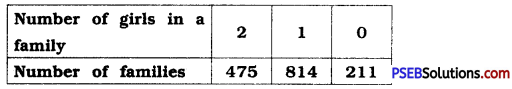Compute the probability of a family, chosen at random, having
(i) 2 girls
(ii) 1 girl
(iii) No girl. Also check whether the sum of these probabilities is 1.
Here, the total number of families is 1500.
Hence, the total number of trials = 1500

(i) Let event A denote the event that the family chosen at random is having 2 girls.
Then, the number of trials when event A occured is 475.
∴ P(A) = $$\frac{\text { No. of trials in which event A occured }}{\text { The total number of trials }}$$
= $$\frac{475}{1500}$$
= $$\frac{19}{60}$$

(ii) Let event B denote the event that the family chosen at random is having 1 girl.
Then, the number of trials when event B occured is 814.
∴ P(B) = $$\frac{\text { No. of trials in which event B occured }}{\text { The total number of trials }}$$
= $$\frac{814}{1500}$$
= $$\frac{407}{750}$$

(iii) Let event C denote the event that the family chosen at random Is having no girl.
Then, the number of trials when event C occured is 211.
∴ p(C) = $$\frac{\text { No. of trials in which event } \mathrm{C} \text { occured }}{\text { The total number of trials }}$$
= $$\frac{211}{1500}$$
Now,
P(A) + P(B) + P(C) = $$\frac{19}{60}+\frac{407}{750}+\frac{211}{1500}$$
= $$\frac{475+814+211}{1500}$$
= $$\frac{1500}{1500}$$
= 1Question 3.
Refer to sum no. 5 of “Sums to Enrich ‘Remember’” in chapter 14. Find the probability that a student of the class was born in August.
From the Bar graph in the sum which is referred here, we get the following information:

Total number of students = 40 and the number of students born in August = 6.
Hence, if event A denotes the event that a student of the class is born in August, then the number of trials when event A occured is 6 and the total number of trials is 40.
∴ P(A) = $$\frac{\text { No. of trials in which event A occured }}{\text { The total number of trials }}$$
= $$\frac{6}{40}$$
= $$\frac{3}{20}$$

Question 4.
Three coins are tossed simultaneously 200 times with the following frequencies of different outcomes: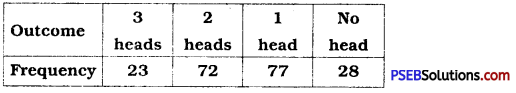If the three coins are simultaneously tossed again, compute the probability of 2 heads coming up.
Here, the total number of trials = 200. If event A denotes the event that 2 heads come up, then the number of trials when event A occured is 72.
∴ P(A) = $$\frac{\text { No. of trials in which event A occured }}{\text { The total number of trials }}$$
= $$\frac{72}{200}$$
= $$\frac{9}{25}$$Question 5.
An organisation selected 2400 families at random and surveyed them to determine a relationship between income level and the number of vehicles in a family. The information gathered is listed in the table below :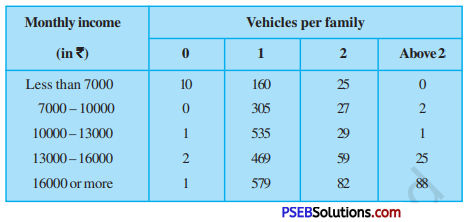Suppose a family is chosen. Find the probability that the family chosen is ( i ) earning ? 10000- ? 13000 per month and owning exactly 2 vehicles.
(ii) earning ₹ 16000 or more per month and owning exactly 1 vehicle.
(iii) earning less than ₹ 7000 per month and does not .own any vehicle.
(iv) earning ₹ 13000 – ₹ 16000 per month and owning more than 2 vehicles.
(v) owning not more than 1 vehicle.
Here, the total number of families is 2400. Hence, the total number of trials = 2400

(i) Let event A denote the event that the family is earning ₹ 10000 – ₹ 13000 per month and owning exactly 2 vehicles.
Then, the number of trials when event A occured = 29.
∴ P(A) = $$\frac{\text { No. of trials in which event A occured }}{\text { The total number of trials }}$$
= $$\frac{29}{2400}$$(ii) Let event B denote the event that the family is earning ₹ 16000 or more per month and owning exactly 1 vehicle.
Then, the number of trials when even B occured = 579.
∴ P(B) = $$\frac{\text { No. of trials in which event B occured }}{\text { The total number of trials }}$$
= $$\frac{579}{2400}$$
= = $$\frac{193}{800}$$

(iii) Let event C denote the event that the family is earning less than ₹ 7000 per month and does not own any vehicle.
Then, the number of trials when event C occured = 10.
∴ P(C) = $$\frac{\text { No. of trials in which event C occured }}{\text { The total number of trials }}$$
= $$\frac{10}{2400}$$
= = $$\frac{1}{240}$$

(iv) Let event D denote the event that the family is earning ? 13000 -? 16000 per month and is owning more than 2 vehicles. Then, the number of trials when event D occured = 25.
∴ P(D) = $$\frac{\text { No. of trials in which event D occured }}{\text { The total number of trials }}$$
= $$\frac{25}{2400}$$
= $$\frac{1}{96}$$

(v) Let event E denote the event that the family is owning not more than 1 vehicle, i.e., 1 vehicle or no vehicle.
Then, the number of trials when event E occured.
= 10 + 160 + 0 + 305 + 1 + 535 + 2 + 469 + 1 + 579 = 2062
∴ P(E) = $$\frac{\text { No. of trials in which event E occured }}{\text { The total number of trials }}$$
= $$\frac{2062}{2400}$$
= $$\frac{1031}{1200}$$Question 6.
Refer to table 7 of sum no. 7 in “Sums to Enrich ‘Remember’” in chapter 14.
(i) Find the probability that a student obtained less than 20 marks in the mathematics test.
(ii) Find the probability that a student obtained marks 60 or above.
According to the table referred here, the total number of students = 90.
Hence, the total number of trials = 90.
(i) According to the same table, the number of students who obtained less than 20 marks in the mathematics test is 7. So, if the event that a student obtained less than 20 marks in mathematics test is called event A, then the number of trials when event A occured is 7.
∴ P(A) = $$\frac{\text { No. of trials in which event A occured }}{\text { The total number of trials }}$$
= $$\frac{7}{90}$$

(ii) Let event B denote the event that a student obtained 60 or more marks. Then, , according to the same table, the number of trials when event B occured = 15 + 8 = 23.
∴ P(B) = $$\frac{\text { No. of trials in which event B occured }}{\text { The total number of trials }}$$
= $$\frac{23}{90}$$

Question 7.
To know the opinion of the students about the subject statistics, a survey of 200 students was conducted. The data is recorded in the following table:

 Opinion Number of students Like 135 Dislike 65

Find the probability that a student chosen at random
(i) Likes statistics,
(ii) Does not like it.
Here, the total number of students = 200.
Hence, the total number of trials = 200.(i) Let event A denote the event that a student likes statistics.
Then, the number of trials when event A occured = 135
∴ P(A) = $$\frac{\text { No. of trials in which event A occured }}{\text { The total number of trials }}$$
= $$\frac{135}{200}$$
= $$\frac{27}{40}$$

(ii) Let event B denote the event that a student does not like statistics. Then, the number of trials when event B occured = 65.
∴ P(B) = $$\frac{\text { No. of trials in which event B occured }}{\text { The total number of trials }}$$
= $$\frac{65}{200}$$
= $$\frac{13}{40}$$

Question 8.
Refer to sum no. 2, Exercise 14.2. What is the empirical probability that an engineer lives:
(i) less than 7 km from her place of work ?
(ii) more than or equal to 7km from her place of work ?
(iii) within $$\frac{1}{2}$$km from her place to work?
The total number of observations in the question referred here is 40.
Hence, the total number of trials = 40.

(i) Let event A denote the event that the distance between her residence and the place of work is less than 7 km. Then there are 9 such observations, viz., 5, 3, 2, 3, 6, 5, 6, 2, 3.
Hence, the number of trials when event A occured = 9.
∴ P(A) = $$\frac{\text { No. of trials in which event A occured }}{\text { The total number of trials }}$$
= $$\frac{9}{40}$$(ii) Let event B denote the event that the said distance is 7 km or more than 7 km. Then, all the remaining 31(40-9) observations refer to event B.
Hence, the number of trials when event B occured = 31
∴ P(A) = $$\frac{\text { No. of trials in which event A occured }}{\text { The total number of trials }}$$
= $$\frac{31}{40}$$

(iii) Let event C denote the event that the engineer lives within $$\frac{1}{2}$$ km from her place of work. There is no observation which is $$\frac{1}{2}$$ or less than $$\frac{1}{2}$$.
Hence, the number of trials when event C occured = 0.
∴ P(C) = $$\frac{\text { No. of trials in which event C occured }}{\text { The total number of trials }}$$
= $$\frac{0}{40}$$
= 0

Question 9.
Activity: Note the frequency of two-wheelers, three-wheelers and four-wheelers going past during a time interval, in front of your school gate. Find the probability that any one vehicle out of the total vehicles you have observed is a two-wheeler.
Note: Students should do this Activity themselves.

Question 10.
Activity: Ask all the students in your class to write a 3-digit number. Choose any student from the room at random. What is the probability that the number written by her / him is divisible by 3 ? Remember that a number is divisible by 3, if the sum of its digits is divisible by 3.
Note: Students should do this Activity themselves.

Question 11.
Eleven bags of wheat flour, each marked 5 kg, actually contained the following weights of flour (in kg) :
4.97 5.05 5.08 5.03 5.00 5.06 5.08 4.98 5.04 5.07 5.00
Find the probability that any of these bags chosen at random contains more than 5 kg of flour.
The total number of bags = 11.
Hence, the total number of trials = 11.
Let event A denote the event that a bag contains more than 5 kg of flour.
There are 7 bags weighing more than 5 kg.
Their weights (in kg) are 5.05, 5.08, 5.03, 5.06, 5.08, 5.04 and 5.07. Hence, the number of trials when event A occured = 7.
∴ P(A) = $$\frac{\text { No. of trials in which event A occured }}{\text { The total number of trials }}$$
= $$\frac{7}{11}$$Question 12.
In sum no. 5, Exercise 14.2, you were asked to prepare a frequency distribution table, regarding the concentration of sulphur dioxide in the air in parts per million of a certain city for 30 days. Using this table, find the probability of the concentration of sulphur dioxide in the interval 0.12 – 0.16 on any of these days.
In sum no. 5, Exercise 14.2, total number of days is 30.
Hence, the total number of trials = 30.
In the table prepared there, we see that the frequency of class 0.12 – 0.16 is 2.
Hence, during 2 days the concentration of sulphur dioxide (in ppm) was in the interval 0.12 – 0.16.
Let event A denote the event that the concentration of sulphur dioxide (in ppm) is in the interval 0.12 – 0.16.
Hence, the number of trials when event A occured = 2.
∴ P(A) = $$\frac{\text { No. of trials in which event A occured }}{\text { The total number of trials }}$$
= $$\frac{2}{30}$$
= $$\frac{1}{15}$$

Question 13.
In sum no. 1, Exercise 14.2, you were asked) to prepare a frequency distribution table regarding the blood groups of 30 students of a class. Use this table to determine the probability that a student of this class, selected at random, has blood group AB.
In sum no. 1, Exercise 14.2, the total number of students is 30.
Hence, the total number of trials = 30.
Let event A denote the event that a student has blood group AB. The number of students having blood group AB is 3.
Hence, the number of trials when event A occured = 3.
∴ P(A) = $$\frac{\text { No. of trials in which event A occured }}{\text { The total number of trials }}$$
= $$\frac{3}{30}$$
= $$\frac{1}{10}$$

PSEB 9th Class Maths MCQ Chapter 14 Statistics

Punjab State Board PSEB 9th Class Maths Book Solutions Chapter 14 Statistics MCQ Questions with Answers.

PSEB 9th Class Maths Chapter 14 Statistics MCQ Questions

Answer each question by selecting the proper alternative from those given below each question to make the statement true:

Question 1.
The marks scored by Kavya in 10 tests of Mathematics are 35, 18, 41, 24, 45, 10, 28, 32, 40, 15. Then, the range of the data is …………….. .
A. 45
B. 10
C. 35
D. 28.8
C. 35

Question 2.
The average of the observations 3, 4, 5, 8, 12, 10, 13, 16, 18, 11 is …………………. .
A. 100
B. 10
C. 18
D. 3
B. 10Question 3.
The mean of first five odd natural numbers is ……………….. .
A. 3
B. 5
C. 4
D. 25
B. 5

Question 4.
The mean of first four even natural numbers is ……………….. .
A. 5
B. 10
C. 20
D. 4
A. 5Question 5.
The mean of first five prime numbers is
A. 28
B. 2.8
C. 5.6
D. 1.4
C. 5.6

Question 6.
If the mean of 2x, 5, 3x, 12, 5x, 17 and 6 is 20, then x = ………………….. .
A. 10
B. 20
C. 15
D. 40
A. 10Question 7.
The mean of the following distribution is ………………. .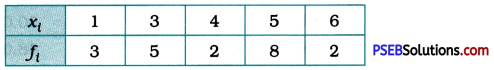A. 3.9
B. 7.8
C. 78
D. 39
A. 3.9

Question 8.
If the mean of 12, 13, x, 17, 18 and 20 is 16, then x = ………………. .
A. 8
B. 4
C. 16
D. 32
C. 16Question 9.
For a given frequency distribution, n = 20 and Σf<sub>i</sub>x<sub>i</sub> = 140, then X̄ = ………………… .
A. 20
B. 14
C. 7
D. 28
C. 7

Question 10.
The mean of $$\frac{2}{5},$$, $$\frac{5}{7},$$, $$\frac{3}{5},$$ and $$\frac{2}{7},$$ is ……………… .
A. $$\frac{1}{2},$$
B. $$\frac{3}{5},$$
C. $$\frac{5}{7},$$
D. 2
A. $$\frac{1}{2},$$Question 11.
The median of 14, 6, 2, 13, 9, 15 and 12 is …………………. .
A. 12
B. 10
C. 2
D. 15
A. 12

Question 12.
The median of 21, 17, 13, 33, 19, 23 is ………………… .
A. 21
B. 20
C. 33
D. 19
B. 20

PSEB 9th Class Maths Solutions Chapter 14 Statistics Ex 14.4

Punjab State Board PSEB 9th Class Maths Book Solutions Chapter 14 Statistics Ex 14.4 Textbook Exercise Questions and Answers.

PSEB Solutions for Class 9 Maths Chapter 14 Statistics Ex 14.4

Question 1.
The following number of goals were scored by a team in a series of 10 matches:
2, 3. 4, 5, 0. 1, 3, 3, 4, 3
Find the mean, median and mode of these scores.
Here, n = 10.
Mean X̄ = $$\frac{\Sigma x_{i}}{n}$$
= $$\frac{2+3+4+5+0+1+3+3+4+3}{10}$$
= $$\frac{28}{10}$$
= 2.8
Thus, the mean of the given scores is 2.8 goals.

Arranging the observations in the ascending order, we get:
0, 1, 2, 3, 3, 3, 3, 4, 4, 5
Since n = 10 is an even number, $$\frac{n}{2}$$ = 5 and $$\frac{n}{2}$$ + 1 = 6.

Median M
= $$\frac{\left(\frac{n}{2}\right) \text { th observation }+\left(\frac{n}{2}+1\right) \text { th observation }}{2}$$
= $$\frac{5 \text { th } \text { observation }+6 \text { th } \text { observation }}{2}$$
= $$\frac{3+3}{2}$$ = 3
Thus, the median of the given scores is 3 goals.
In the given data, observation 3 occurs most frequently (4 times). Hence, the mode of the data is 3 goals.Question 2.
In a mathematics test given to 15 students, the following marks (out of 100) are recorded :
41, 39, 48, 52, 46, 62, 54, 40, 96, 52, 98, 40, 42, 52, 60
Find the mean, median and mode of this data.
Here, n = 15.
Mean X̄ = $$\frac{\Sigma x_{i}}{n}$$
= $$\begin{gathered} 41+39+48+52+46+62+54+40 \\ +96+52+98+40+42+52+60 \\ \hline 15 \end{gathered}$$
= $$\frac{822}{15}$$ = 54.8
Thus, the mean of the data is 54.8 marks.
Arranging the observations in the ascending order, we get:
39, 40, 40, 41, 42, 46, 48, 52, 52, 52, 54, 60, 62, 96, 98
Here, n = 15 is an odd number.
Median M = $$\left(\frac{n+1}{2}\right)$$th observation
= $$\left(\frac{15+1}{2}\right)$$th observation
= 8 th observation
= 52
Thus, the median of the data is 52 marks.
In the given data, observation 52 occurs most frequently (3 times). Hence, the mode of the data is 52 marks.Question 3.
The following observations have been arranged in ascending order. If the median of the data is 63, find the value of x.
29, 32, 48, 50, x, x + 2, 72, 78, 84, 95
Here, the median = 63 and n = 10.
∴ $$\frac{n}{2}$$ = 5 and $$\frac{n}{2}$$ + 1 = 6

Median M
= $$\frac{\left(\frac{n}{2}\right) \text { th observation }+\left(\frac{n}{2}+1\right) \text { th observation }}{2}$$
∴ 63 = $$\frac{5 \text { th } \text { observation }+6 \text { th } \text { observation }}{2}$$
∴ 63 = $$\frac{(x)+(x+2)}{2}$$
∴63 × 2 = x + x + 12
∴126 = 2x + 2
∴ 2x = 124
∴ x = 62Question 4.
Find the mode of 14, 25, 14, 28, 18, 17, 18, 14, 23, 22, 14, 18.
Here, just by simple observation, it is clearly seen that observation 14 occurs most frequently, i.e., 4 times.
Hence, the mode of the data is 14.

Question 5.
Find the mean salary of 60 workers of a factory from the following table: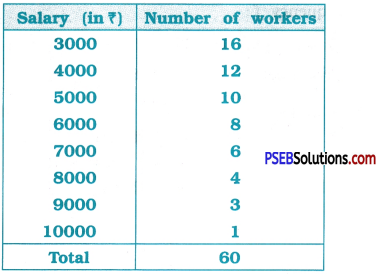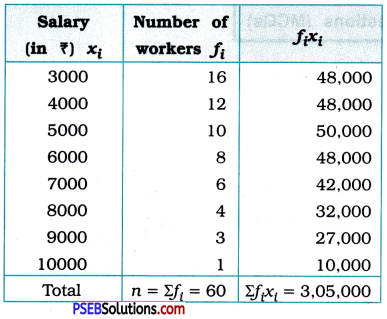Mean X̄ = $$\frac{\Sigma f_{i} x_{i}}{n}$$
=  = $$\frac{3,05,000}{60}$$ = 5083.33
Thus, the mean salary is ₹ 5083.33.Question 6.
Give one example of a situation in which
(i) the mean is an appropriate measure of central tendency.
For the students studying in the same class, usually their level of knowledge and understanding would be more or less equal. There would be a few student having this level low and there would be a few students having this level high. Their level of knowledge and understanding would be reflected in the marks scored by them at an examination. Hence, the mean of marks scored by them at an examination is an appropriate measure of central tendency.

(ii) the mean is not an appropriate measure of central tendency but the median is an appropriate measure of central tendency.
If we consider the monthly income of the people of certain region, the mean is not an appropriate measure of central tendency but the median is an appropriate measure of central tendency.

PSEB 9th Class Maths Solutions Chapter 14 Statistics Ex 14.3

Punjab State Board PSEB 9th Class Maths Book Solutions Chapter 14 Statistics Ex 14.3 Textbook Exercise Questions and Answers.

PSEB Solutions for Class 9 Maths Chapter 14 Statistics Ex 14.3

Question 1.
A survey conducted by an organisation for the cause of illness and death among the women between the ages 15-44 (in years) worldwide, found the following figures (in %):

 Causes Female fatality rate (%) 1. Reproductive health conditions 31.8 2. Neuropsychiatric conditions 25.4 3. Injuries 12.4 4. Cardiovascular conditions 4.3 5. Respiratory conditions 4.1 6. Other causes 22.0

(i) Represent the information given above graphically.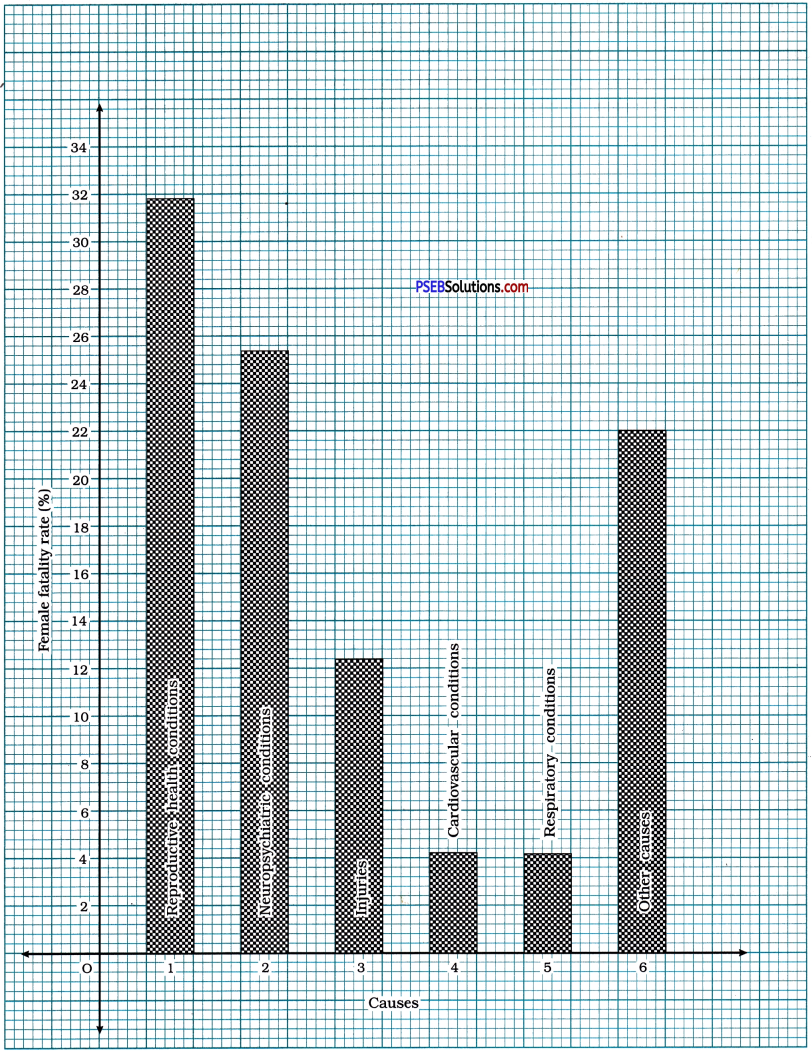(ii) Which condition is the major cause of women’s ill health and death worldwide?
‘Reproductive health conditions’ is the major cause of womens ill health and death worldwide.(iii) Try to find out, with the help of your teacher, any two factors which play a major role in the cause in (ii) above being the major cause.
‘Malnutrition’ and ‘Lack of necessary medical facilities’ can be considered as two other factors which play a major role in female fatality.

Question 2.
The following data on the number of girls (to the nearest ten) per thousand boys in different sections of Indian society is given below:

 Section Number of girls per thousand bays Scheduled Caste (SC) 940 Scheduled Tribe (ST) 970 Non-SC/ST 920 Backward districts 950 Non-backward districts 920 Rural 930 Urban 910

(i) Represent the information above by a bar graph.
(ii) In the classroom discuss what conclusions can be arrived at from the graph.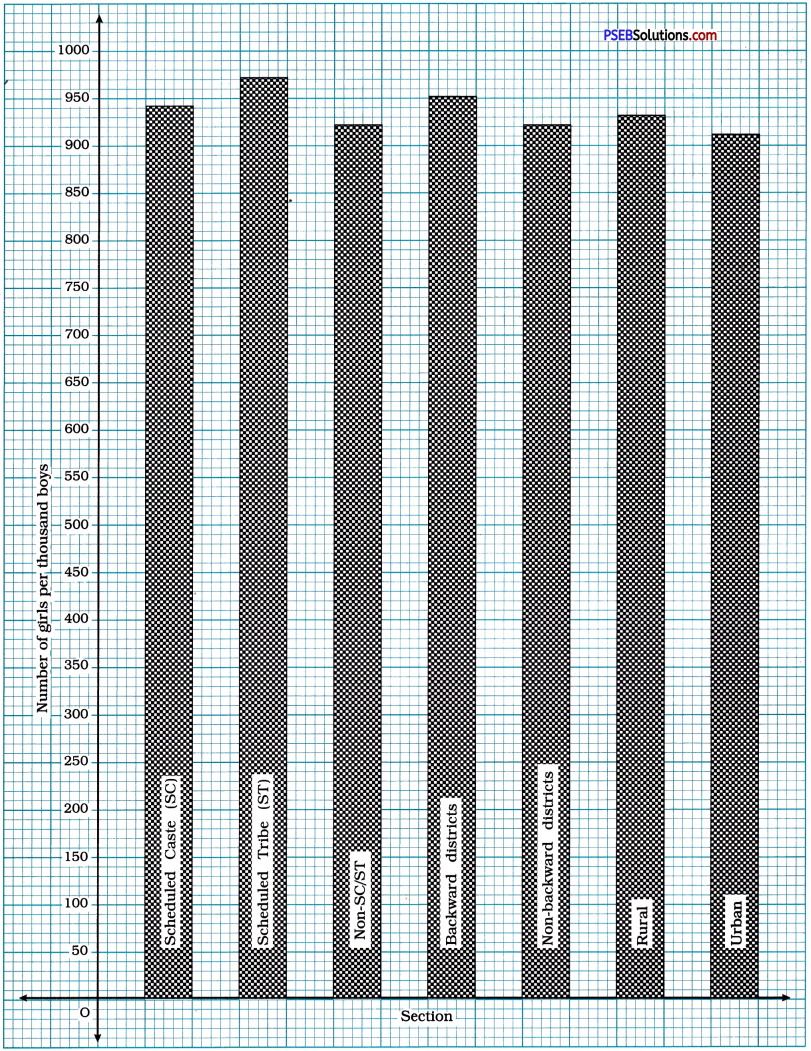Question 3.
Given below are the seats won by different political parties in the polling outcome of a state assembly elections:(i) Draw a bar graph to represent the polling results.
Seats won by different political parties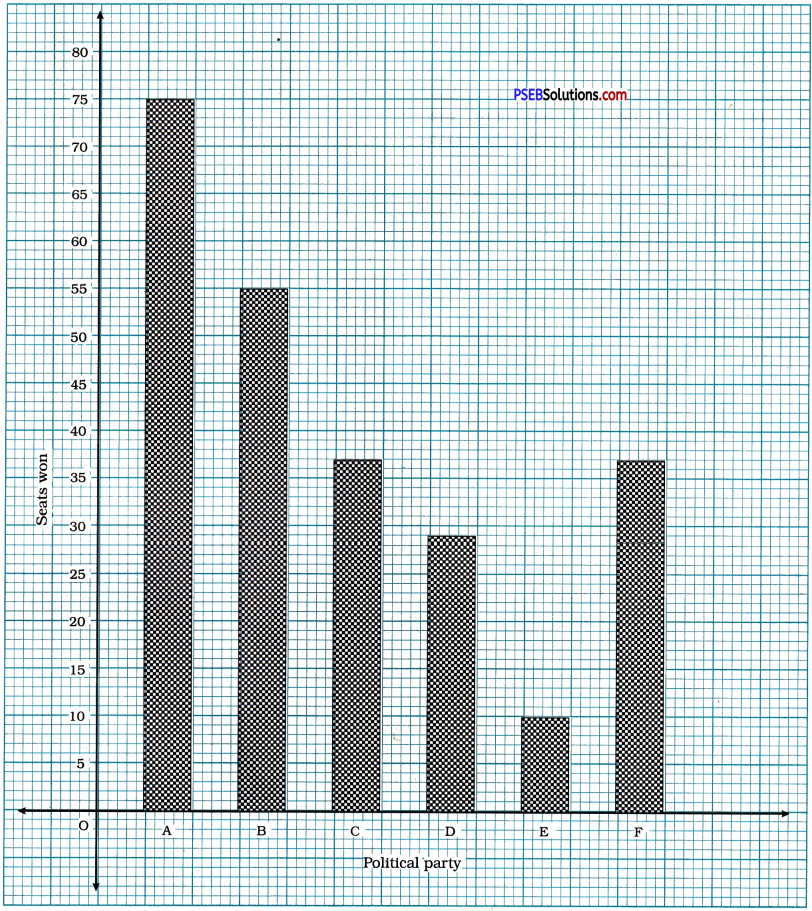(ii) Which political party won the maximum number of seats?
Political party: A won the maximum number of seats.Question 4.
The length of 40 leaves of a plant are measured correct to one millimetre, and the obtained data is represented in the following table:

 Length (in mm) Number of leaves 118-126 3 127-135 5 136-144 9 145-153 12 154-162 .5 163-171 4 172-180 2

(i) Draw a histogram to represent the given data. [Hint: First make the class intervals continuous.]
(ii) Is there any other suitable graphical representation for the same data?
(iii) Is it correct to conclude that the maximum number of leaves are 153 mm long? Why?
Making the class intervals continuous, we get the following table:

 Length (in mm) Number of leaves 117.5-126.5 3 126.5- 135.5 5 135.5-144.5 9 144.5-153.5 12 153.5- 162.5 5 162.5-171.5 4 171.5-180.5 2

(i) Length of leaves in millimetre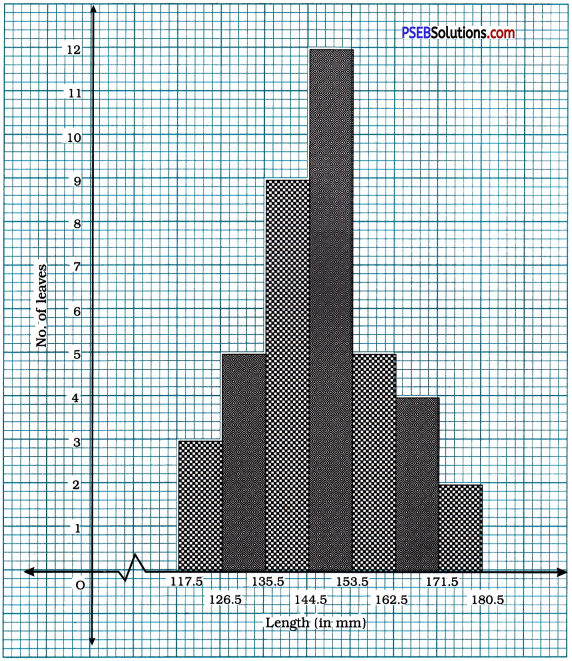(ii) Yes. The given data can also be represented by ‘Frequency polygon’.

(iii) It is not correct to conclude that the maximum number of leaves are 153 mm long, because even if the frequency of class 145-153 is 12, we do not have the information about the length of each of those 12 leaves individually.Question 5.
The following table gives the life times of 400 neon lamps:

 Life time (in hours) Number of lamps 300 – 400 14 400 – 500 56 500 – 600 60 600 – 700 86 700 – 800 74 800 – 900 62 900 – 1000 48

(i) Represent the given information with the help of a histogram.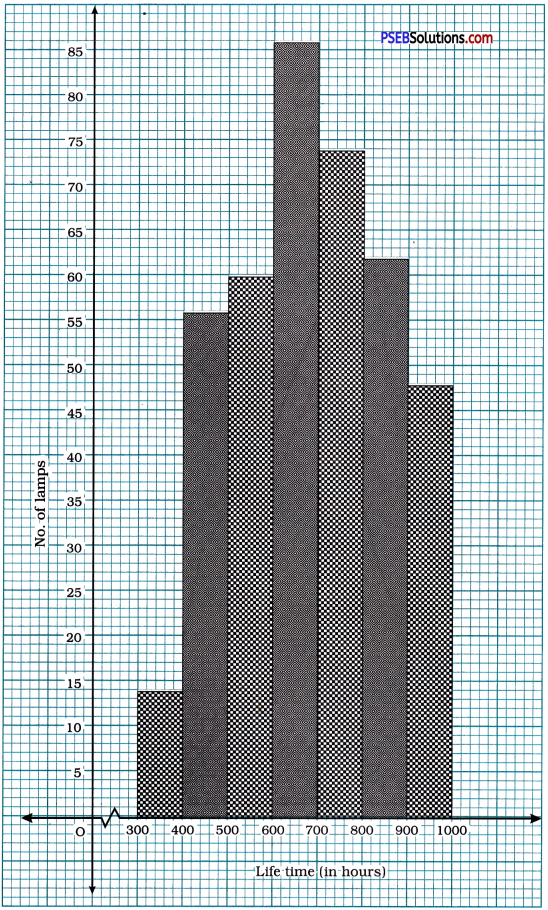(ii) How many lamps have a life time of 700 hours or more than 700 hours ?
The-frequencies of classes 700-800, 800-900 and 900-1000 are 74, 62 and 48 respectively.
Hence, the life time of 184 (74 + 62 + 48) lamps is 700 hours or more than 700 hours.

Question 6.
The following table gives the distribution of students of two sections according to the marks obtained by them: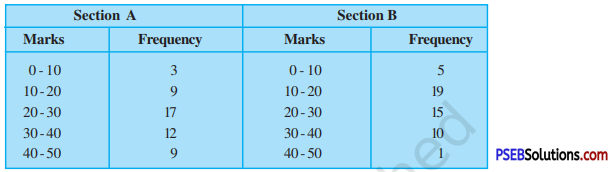Represent the marks of the students of both the sections on the same graph by two frequency polygons. From the two polygons compare the performance of the two sections.
To draw the frequency polygons of both the sections, we find the class marks of each class and prepare the following tables:

Section A

 Marks Class mark Frequency 0-10 5 3 10-20 15 9 20-30 25 17 30-40 35 12 40-50 45 9

Section B

 Marks Class mark Frequency 0-10 5 5 10-20 15 19 20-30 25 15 30-40 35 10 40-50 45 1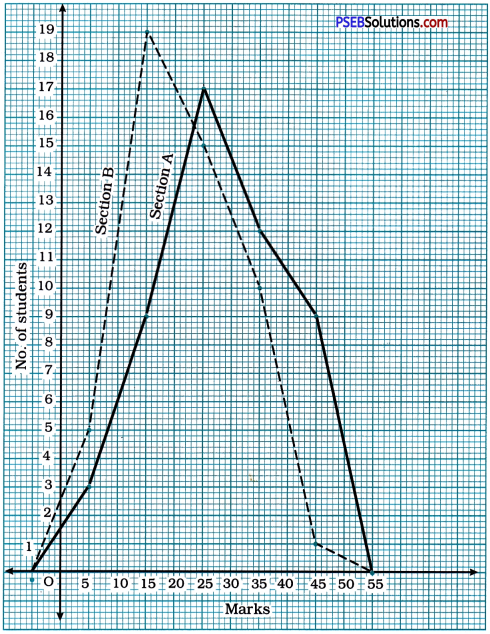Comparing the performance of both the sections from the frequency polygons, we observe that the performance of students of section A is better than the performance of students of section B.Question 7.
The runs scored by two teams A and B on the first 60 balls in a cricket match are given below:

 Number of balls Team A Team B 1-6 2 5 7-12 1 6 13-18 8 2 19-24 9 10 25-30 4 5 31-36 5 6 37-42 6 3 43-48 10 4 49-54 6 8 55-60 2 10

Represent the data of both the teams on the same graph by frequency polygons.
[Hint: First make the class intervals continuous.]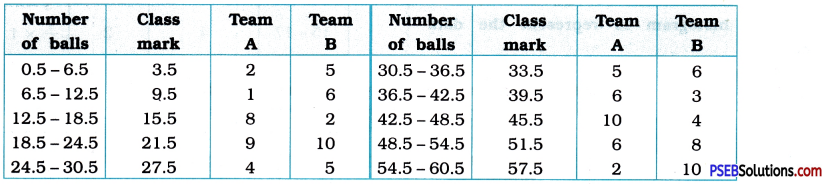Number of runs made by Team A and Team B in first 60 balls.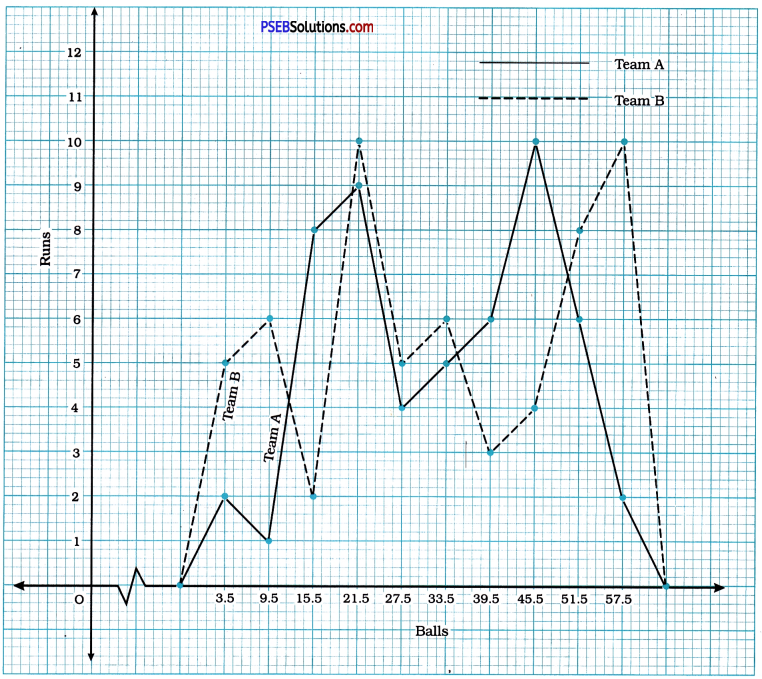Question 8.
A random survey of the number of children of various age groups playing in a park was found as follows:

 Age (in years) Number of children 1-2 5 2-3 3 3-5 6 5-7 12 7-10 9 10-15 10 15-17 4

Draw a histogram to represent the data above.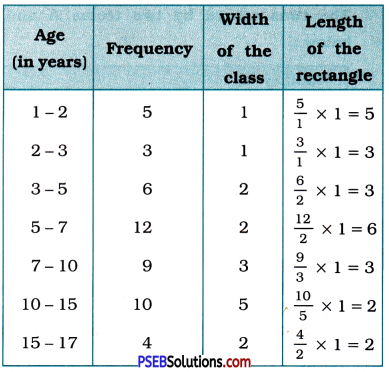Children of various age groups playing in a park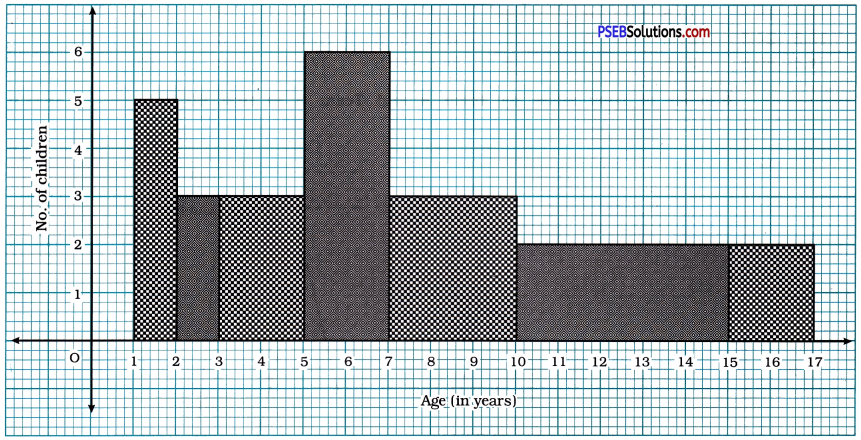Question 9.
100 surnames were randomly picked up from a local telephone directory and a frequency distribution of the number of letters in the English alphabet in the surnames was found as follows:

 Number of letters Number of surnames 1-4 6 4-6 30 6-8 44 8-12 16 12-20 4

(i) Draw a histogram to depict the given information.
(ii) Write the class interval in which the maximum number of surnames lie.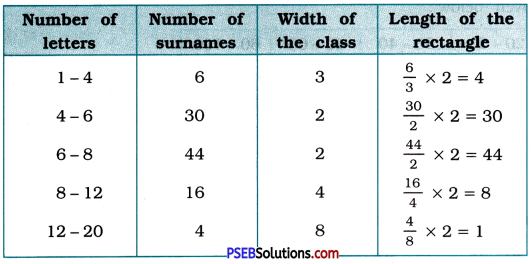(i) Information regarding the number of surnames having given number of letters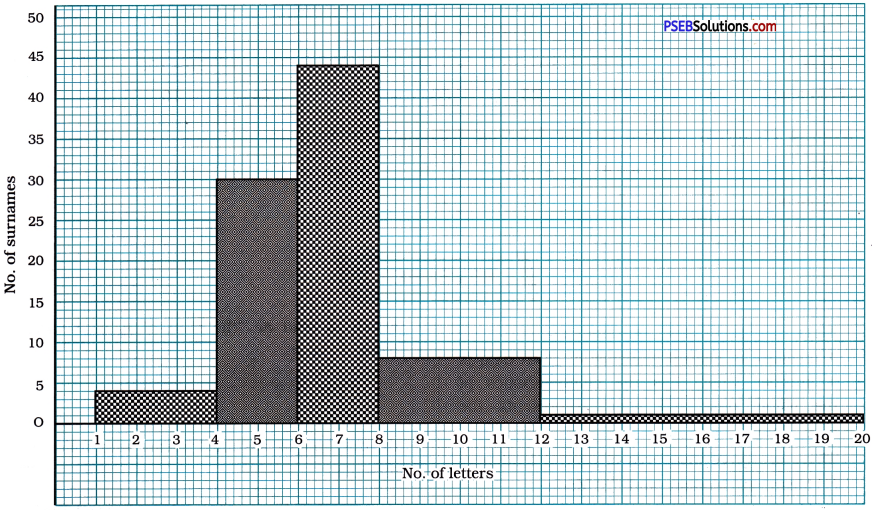(ii) Write the class interval in which the maximum number of surnames lie.
The maximum number of surnames lie in the class interval 6-8.

PSEB 9th Class Maths Solutions Chapter 13 Surface Areas and Volumes Ex 13.9

Punjab State Board PSEB 9th Class Maths Book Solutions Chapter 13 Surface Areas and Volumes Ex 13.9 Textbook Exercise Questions and Answers.

PSEB Solutions for Class 9 Maths Chapter 13 Surface Areas and Volumes Ex 13.9

Question 1.
A wooden bookshelf has external dimensions as follows : Height =110 cm, Depth = 25 cm, Breadth = 85 cm (see the given figure). The thickness of the plank is 5 cm everywhere. The external faces are to be polished and the inner faces are to be painted. If the rate of polishing is 20paise per cm2 and the rate of painting is 10 paise per cm2, find the total expenses required for polishing and painting the surface of the bookshelf.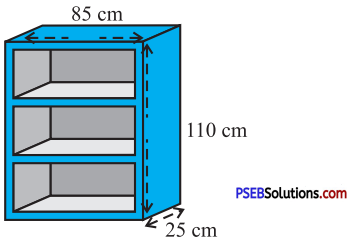Outer faces to be polished:

• One face on back side of the bookshelf, measuring 110 cm × 85 cm.
• Two faces on the sides, each of those measuring 110 cm × 25 cm.
• The top and the base, each of those measuring 85 cm × 25 cm.
• Two vertical strips on the front side, each of those measuring 110 cm × 5 cm.
• Four horizontal strips on the front side, each of those measuring 75 cm × 5 cm.

Thus, total area of region to be polished
= [(110 × 85) + 2(110 × 25) + 2 (85 × 25) + 2(110 × 5) + 4(75 × 5)] cm2
= (9350 + 5500 + 4250 + 1100+ 1500) cm2
= 21700 cm2
20 paise per cm2 = ₹ 0.20 per cm2
Cost of polishing 1 cm2 region = ₹ 0.20
∴ Cost of polishing 21700 cm2 region
= ₹ (21700 × 0.20)
= ₹ 4340Inner faces to be painted:

• Two faces on the sides each of those measuring 90 cm × 20 cm.
• Two faces each of two shelves, the top face and the bottom face, in all six face, each of those measuring 75 cm × 20 cm.
• Face on the back side, measuring 90 cm × 75 cm.

Thus, total area of the region to be painted
= [2 (90 × 20) + 6 (75 × 20) + (90 × 75)] cm2
= (3600 + 9000 + 6750) cm2
= 19350 cm2
10 paise per cm2 = ₹0.10 per cm2
Cost of painting 1 cm2 region = ₹ 0.10
∴ Cost of painting 19350 cm2 region = ₹ (19350 × 0.10) = ₹ 1935
Then, the total expense of polishing and painting = ₹ 4340 + ₹ 1935 = ₹ 6275Question 2.
The front compound wall of a house is decorated by wooden spheres of diameter 21 cm, placed on small supports as shown in the given figure. Eight such spheres are used for this purpose, and are to be ‘ painted silver. Each support is a cylinder of radius 1.5 cm and height 7 cm and is to be painted black. Find the cost of paint required if silver paint costs 25 paise per cm2 and black paint costs 5 paise per cm2.For each wooden sphere,
radius r = $$\frac{\text { diameter }}{2}$$ = $$\frac{21}{2}$$ cm
Curved surface area of 1 sphere
= 4πr2
= 4 × $$\frac{22}{7}$$ × $$\frac{21}{2}$$ × $$\frac{21}{2}$$ cm2
= 1386 cm2
For each cylindrical support, radius r = 1.5 cm and height h = 7 cm.
Area of top of cylindrical support
= πr2
= $$\frac{22}{7}$$ × 1.5 × 1.5 cm2
= 7.07 cm2 (approx.)
Hence, the area of each sphere to be painted silver = 1386 cm2 – 7.07 cm2 = 1378.93 cm2
∴ Total area of eight spheres to be painted silver = 1378.93 cm2 × 8 = 11031.44 cm2
25 paise per cm2 = ₹ 0.25 per cm2
Cost of painting silver in 1 cm2 region = ₹ 0.25
∴ Cost of painting silver in 11031.44 cm2 region
= ₹ (11031.44 x 0.25)
= ₹ 2757.86 (approx.)
Curved surface area of 1 cylindrical support
= 2πrh
= 2 × $$\frac{22}{7}$$ × 1.5 × 7 cm
= 66 cm2
∴ Total area of eight cylindrical supports to be painted black = 66 cm2 × 8 = 528 cm2
5 paise per cm2 = ₹ 0.05 per cm2
Cost of painting black in 1 cm2 region = ₹ 0.05
∴ Cost of painting black in 528 cm2 region = ₹ (528 × 0.05)
= ₹ 26.40
Thus, the total cost of painting = ₹ 2757.86 + ₹ 26.40
= ₹ 2784.26 (approx.)Question 3.
The diameter of a sphere is decreased by 25 %. By what per cent does its curved surface area decrease?
Suppose, the initial diameter of the sphere is d units and radius is r units.
∴ d = 2r
Original curved surface area of the sphere
= 4πr2
= π (4r2)
= π (2r)2
= πd2 unit2
Now, the diameter of the sphere is reduced by 25 %. Hence, the new diameter of the sphere is 0.75d units.
New curved surface area of the sphere
= π (diameter)
= π (0.75d)2 unit2
= 0.5625 πd2 unit2
∴ The decrease in the curved surface area of the sphere = πd2 – 0.5625 πd2
= 0.4375 πd2 unit2
∴Percentage decrease in the curved surface area of the sphere = $$\frac{0.4375 \pi d^{2}}{\pi d^{2}}$$ × 100 = 43.75 %
Thus, when the diameter of a sphere is decreased by 25 %, its curved surface area decreases by 43.75 %.

PSEB 9th Class Physical Education Book Solutions Guide in Punjabi English Medium

Punjab State Board Syllabus PSEB 9th Class Physical Education Book Solutions Guide Pdf in English Medium and Punjabi Medium are part of PSEB Solutions for Class 9.

PSEB 9th Class Physical Education Guide | Health and Physical Education Guide for Class 9 PSEB in English Medium

Physical Education Guide for Class 9 PSEB | PSEB 9th Class Physical Education Book Solutions

Physical Education 9th Class PSEB Guide Rules of Games

PSEB 9th Class Physical Education Syllabus and Structure of Question Paper

Time Allowed: 3 Hours

Theory: 50 Marks
Practical: 40 Marks
C.C.E.: 10 Marks
Total: 100 Marks

1. A total of 23 questions would be asked. All questions are compulsory.

2. Q. No. 1 to 10 comprising objective-type questions consisting of 1 mark each. The answer to these questions should not be more than 10 words. (10 × 1 = 10 Marks)

3. Q. No. 11 to 15 comprising questions of 2 marks each. The answer to these questions should be 20 words at least. (5 × 2 = 10 Marks)

4. Q. No. 16 to 20 comprising questions of 3 marks each. The answer to these questions should be 30-50 words. (5 × 3 = 15 Marks)

5. Q. No. 21 to 23 comprising questions of 5 marks, students have to answer 3 questions from the given 5 questions. (3 × 5 = 15 Marks)

• Chapter 1 Physical Education – Its Aim and Objectives.
• Chapter 2 Qualities of Sports and Sportsmanship.
• Chanter 3 Effects of Intoxicants on Sports Performance.
• Chapter 4 First Aid, Strain, Sprain, Dislocation, Fracture and Unconsciousness.
• Chapter 5 Progress of sports in Punjab Since 1947.
• Chapter 6 National Sports.

PSEB 9th Class Physical Education Syllabus (Practical)

Time Allowed: 4 Hours
Practical: 40 Marks

1. Track and Field events (For girls and boys) consisting of 6 marks each. Two events (one track and one field) can be chosen from the following given events:

Track Events: 100-metre race, 200-metre race, 400-metre race, 800-metre race, 3000-metre race and for boys 110-metre hurdle race (Height of Hurdle: 96.4 cm, No. of Hurdles: 10), for girls 100 metre hurdle race (Height of Hurdle: 76.2 cm, No. of Hurdles: 8), 4 × 100 metre (for girls), and 4 × 100 metres (for boys) Relay race. (6 Marks)

Field Events: Hammer Throw, Discus Throw, Javeline Throw, Shotput, Long Jump, High Jump, Triple Jump, Pole Vault. (6 Marks)

2. It is mandatory to choose between the following two groups at least one game each, consisting of 5 marks each.

Games for Boys and Girls:

• Group 1: Handball, Kabaddi, Boxing, Table Tennis, Gymnastics, Football. (5 Marks)
• Group 2: Basketball, Yoga, Gatka, Volleyball, Athletics, Circle Kabaddi. (5 Marks)

3. Marks (maximum marks: 10) would be allotted according to the following order:

• School Representation – 2 Marks
• Zone Representation – 4 Marks
• District Representation – 6 Marks
• State Representation – 8 Marks
• National Representation – 10 Marks

4. Students will make a chart of any Sports/Athletics event. (3 Marks)

5. Viva-voce (5 Marks)

Note: It is mandatory that the student should be in his sports kit during the practical examination.

PSEB 9th Class Welcome Life Book Solutions Guide in Punjabi English Medium

Punjab State Board Syllabus PSEB 9th Class Welcome Life Book Solutions Guide Pdf in English Medium and Punjabi Medium are part of PSEB Solutions for Class 9.

PSEB 9th Class Welcome Life Guide | Welcome Life Guide for Class 9 PSEB in English Medium

Welcome Life Guide for Class 9 PSEB | PSEB 9th Class Welcome Life Book Solutions

PSEB 9th Class Welcome Life Syllabus

Chapter 1 Self Awareness and Self Discipline
Self Awareness and Self Discipline Who am I?, Making your identity, Time Management, Sense of responsibility

Chapter 2 Critical Thinking
My Hobby, Comparing yourself, Proper use of social media

Chapter 3 Interpersonal Relationships
Respecting elders and Loving the young ones, Family tree, Behaviour with peers-1, 2

Chapter 4 Empathy
Managing emotions, Social values and interactions, Unity in diversity, Helping the needy

Chapter 5 Creative Thinking
Constructive thinking, Finding new path, Live performance in public, Making new designs, models, etc.

Chapter 6 Managing Emotions
Identification of emotions, Balancing emotions, Self expression-Diary writing, Positive attitude

Chapter 7 Decision Making
How to take a Decision?, Identifying good and bad habits, Giving the right direction to your dedication

Chapter 8 Problem Solving
Understanding the problem, Technique for solving the problem, Think and find a solution

Chapter 9 Effective Communication
How to interact effectively-1, 2?, Body Language-1, 2

Chapter 10 Coping with Stress
What is stress?, The effects of stress, How to avoid stress?

PSEB 9th Class Maths Solutions Chapter 14 Statistics Ex 14.2

Punjab State Board PSEB 9th Class Maths Book Solutions Chapter 14 Statistics Ex 14.2 Textbook Exercise Questions and Answers.

PSEB Solutions for Class 9 Maths Chapter 14 Statistics Ex 14.2

Question 1.
The blood groups of 30 students of Class VIII are recorded as follows:
A, B, O, O, AB, O, A, O, B, A, O, B, A, O, O,
A, AB, O, A, A, O, O, AB, B, A, O, B, A, B, O.
Represent this data in the form of a frequency distribution table. Which is the most common, and which is the rarest, blood group among these students ?
Frequency distribution table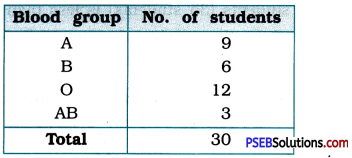From the frequency distribution table, it is very clear that the most common blood group is O and the rarest blood group is AB.Question 2.
The distance (in km) of 40 engineers from their residence to their place of work were found as follows: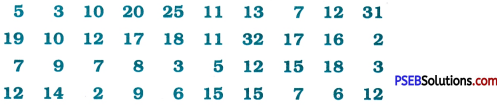Construct a grouped frequency distribution table with class size 5 for the data given above taking the first interval as 0-5 (5 not included). What main features do you observe from this tabular representation?
Grouped frequency distribution table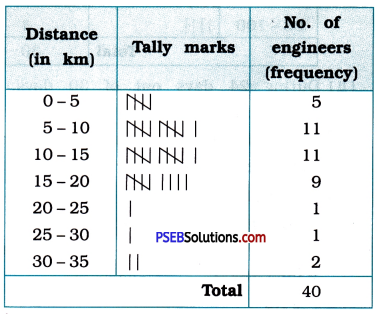From the frequency distribution table, we can conclude that for the majority of engineers, s i.e., 31 engineers, the distance from their residence to their place to work is 5 km or more than 5 km but less than 20 km. For some engineers, i.e., 5 engineers, this distance is less than 5 km. Still, for some engineers, i.e., 4 engineers, this distance is 20 km or more than 20 km but less than 35 km.Question 3.
The relative humidity (in %) of a certain city for a month of 30 days was as follows: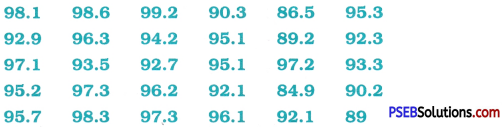(i) Construct a grouped frequency distribution table with classes 84-86, 86 – 88, etc.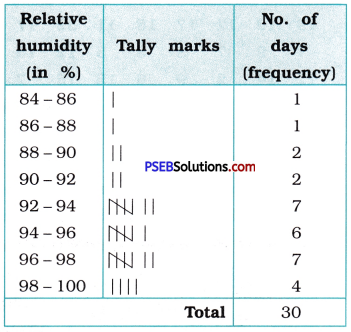(ii) Which month or season do you think this data is about ?
During 24 days out of 30 days, the relative humidity is 92 % or more than 92 %. This suggests that the data must have been collected during Monsoon.

(iii) What is the range of this data ?
Range of the data
= The greatest observation – The least observation
= 99.2 – 84.9
= 14.3Question 4.
The heights of 50 students, measured to the nearest centimetres, have been found to be as follows: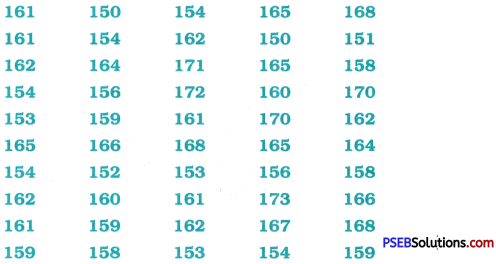(i) Represent the data given above by grouped frequency distribution table, taking the class intervals as 160 – 165, 165-170, etc.
Grouped frequency distribution table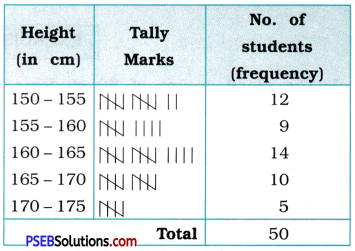(ii) What can you conclude about their heights from the table?
From the above frequency distribution, we can conclude that the height of 70 % students (35 students) is less than 165 cm while the height of only 10 % students (5 students) is 170 cm or more than that.

Question 5.
A study was conducted to find out the concentration of sulphur dioxide in the air in parts per million (ppm) of a certain city. The data obtained for 30 days is as follows :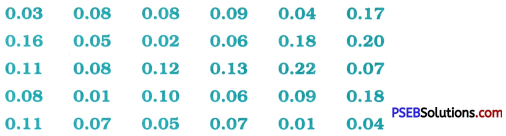(i) Make a grouped frequency distribution table for this data with class intervals as 0.00-0.04, 0.04-0.08, and so on.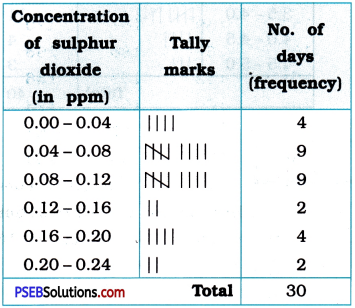(ii) For how many days, was the concentration of sulphur dioxide more than 0.11 parts per million?
The concentration of sulphur dioxide was more than 0.11 ppm for 8 days (2 + 4 + 2).

Question 6.
Three coins were tossed 30 times simultaneously. Each time the number of heads occurring was noted down as follows: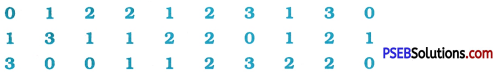Prepare a frequency distribution table for the data given above.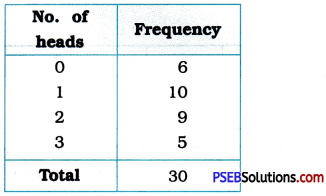Question 7.
The value of π up to 50 decimal places is given below:
3.1415926535897932384626433832795028 8419716939937510
(i) Make a frequency distribution of the digits from 0 to 9 after the decimal point.
Frequency distribution table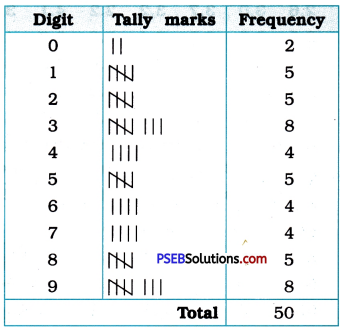(ii) Which are the most and the least frequently occurring digits?
The most frequently occurring digits are 3 and 9 (8 times each) and the least occurring digit is 0 (2 times).Question 8.
Thirty children were asked about the number of hours they watched TV programmes in the previous week. The results were found as follows: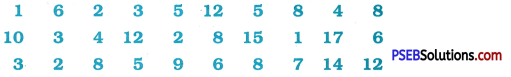(i) Make a grouped frequency distribution table for this data, taking class width 5 and one of the class intervals as 5-10.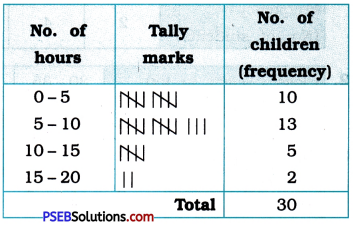(ii) How many children watched television for 15 or more hours a week?
Two children watched television for 15 or more hours a week.Question 9.
A company manufactures car batteries of a Grouped frequency distribution table particular type. The lives (in years) of 40 such batteries were recorded as follows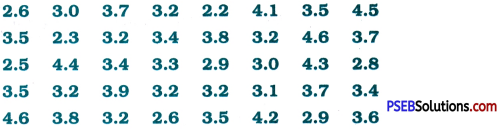Construct a grouped frequency distribution table for this data, using class intervals of size 0.5 starting from the interval 2 – 2.5.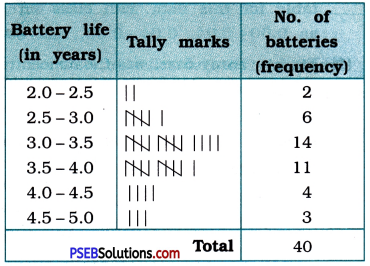PSEB 9th Class Maths Solutions Chapter 14 Statistics Ex 14.1

Punjab State Board PSEB 9th Class Maths Book Solutions Chapter 14 Statistics Ex 14.1 Textbook Exercise Questions and Answers.

PSEB Solutions for Class 9 Maths Chapter 14 Statistics Ex 14.1

Question 1.
Give five examples of data that you can collect from your day-to-day life.
Five examples of data that can be collected from day-to-day life can be given as below:

1. Election results obtained from newspapers or TV
2. The number of different kinds of trees grown in our school.
3. Amounts of invoices of electricity for last one year at our home.
4. The number of students studying in different standards of our school.
5. Percentage of marks scored at last examination by the students in our class.Question 2.
Classify the data in Q. 1 above as primary or secondary data.
Among the five data given as the answer to Q. 1, data no.

Primary data:

• The number of different kinds of trees grown in our school.
• Amounts of invoices of electricity for last one year at our home.
• Percentage of marks scored at last examination by the students in our class.
• primary data which we can collect ourselves.

Secondary data:

• Election results obtained from newspapers or TV
• The number of students studying in different standards of our school.
• Secondary data as they are received from the sources of the newspapers or TV or the office of our school.